# Problem #4: Stack of Polarizers fun and LCD! We know that the relationship between the input...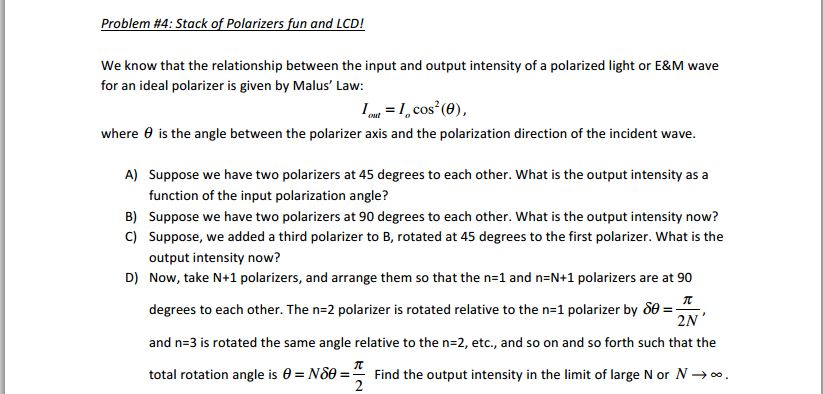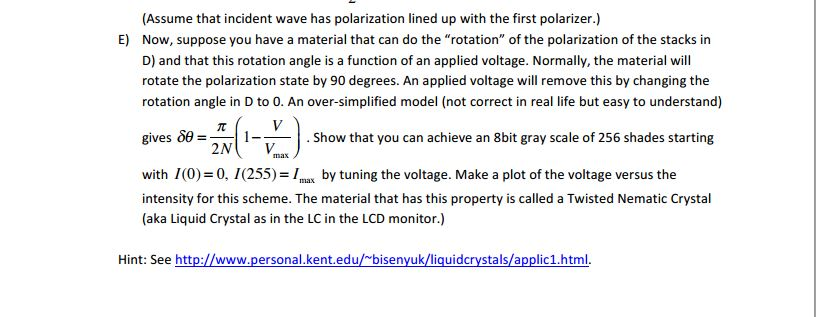Problem #4: Stack of Polarizers fun and LCD! We know that the relationship between the input and output intensity of a polarized light or E&M wave for an ideal polarizer is given by Malus' Law: 1I, cos (0), where θ is the angle between the polarizer axis and the polarization direction of the incident wave. A) Suppose we have two polarizers at 45 degrees to each other. What is the output intensity as a function of the input polarization angle? Suppose we have two polarizers at 90 degrees to each other. What is the output intensity now? Suppose, we added a third polarizer to B, rotated at 45 degrees to the first polarizer. What is the output intensity now? Now, take N+1 polarizers, and arrange them so that the n=1 and n=N+1 polarizers are at 90 B) C) D) degrees to each other. The n-2 polarizer is rotated relative to the n=1 polarizer by #22N and n=3 is rotated the same angle relative to the n-2, etc., and so on and so forth such that the total rotation angle is θ= N39 = Find the output intensity in the limit of large N or N →。。

from the Malus law

(a)

I_out = I_0 cos^2 theta

I_out =I_0 cos^2 45

(b)

I_out = I_0 cos^2 90 = 0

(c)

I_3 =I_0 cos^2 45

=0.5 I_0

I_out put = I_3 cos^2 45 = 0.5 I_0 ( 0.5) = 0.125 I_0

##### Add Answer to: Problem #4: Stack of Polarizers fun and LCD! We know that the relationship between the input...
Similar Homework Help Questions
• ### For the polarizers in the figure, suppose the incident light is linearly polarized, the transmitted intensity...

For the polarizers in the figure, suppose the incident light is linearly polarized, the transmitted intensity (through both polarizers) is 0.15×I0, and the axis of the second polarizer makes an angle θ=50° with the axis of the first polarizer. What is the angle (in degrees) the initial direction of polarization makes with the first polarizer? (Insert the number of degrees without unit.)

• ### Polarizers and a Quarter-Wave PlateA monochromatic laser beam of intensity Io = 659 W/m2 is polarized in the y-direction and propagates in the positive z-direction. This beam is incident upon aquarter-wave plate whose fast axis makes an angle of 45 degrees with the x-axis as shown. Following the quarter-wave plate are two polarizers; the transmissionaxis of the first polarizer is aligned with the x-axis, while the transmission axis of the second polarizer makes an angle of θ1 = 72 degrees with the positive xaxis.1)What...

• ### 5. A plane, linearly polarized light wave, with intensity, Io, is transmitted through a system of perfect linear polarizers (we assume that all light is transmitted in the transmission direction but...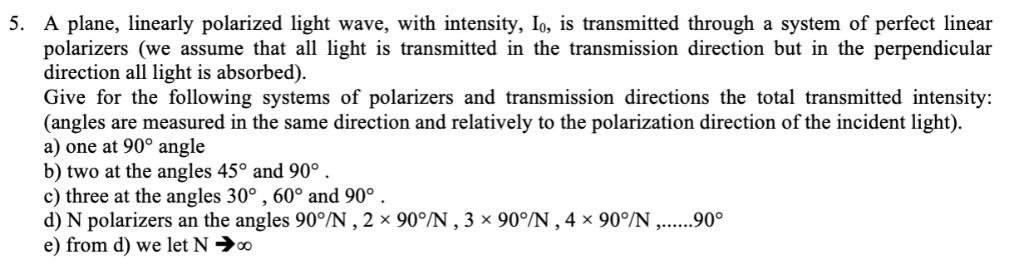5. A plane, linearly polarized light wave, with intensity, Io, is transmitted through a system of perfect linear polarizers (we assume that all light is transmitted in the transmission direction but in the perpendicular direction all light is absorbed). Give for the following systems of polarizers and transmission directions the total transmitted intensity: (angles are measured in the same direction and relatively to the polarization direction of the incident light) a) one at 90° angle b) two at the angles...

• ### Problem 4. Unpolarized light falls on a stack of three polarizers. The second polarizer is rotated...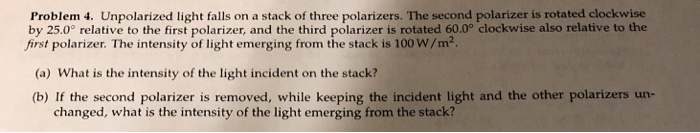Problem 4. Unpolarized light falls on a stack of three polarizers. The second polarizer is rotated clockwise by 25.0° relative to the first polarizer, and the third polarizer is rotated 60.0° clockwise also relative to the first polarizer. The intensity of light emerging from the stack is 100 W/m2 (a) What is the intensity of the light incident on the stack? (b) If the second polarizer is removed, while keeping the incident light and the other polarizers un- changed, what...

• ### Light is entering a polarizer or set of polarizers from the left. Determine the relative intensity...

Light is entering a polarizer or set of polarizers from the left. Determine the relative intensity of the light exiting on the right after passing through all of the polarizers. The dashed vertical lines represent the vertical reference. The solid lines represent either the polarization angle of the light or the transmission angle of the polarizer. CW represents clockwise and CCW represents counterclockwise as viewed from the right looking back to the left along the path of the light. Unpolarized...

• ### (6%) Problem 6: Unpolarized light of intensity lo-1350 W/m2 s incident upon three polarizers. The...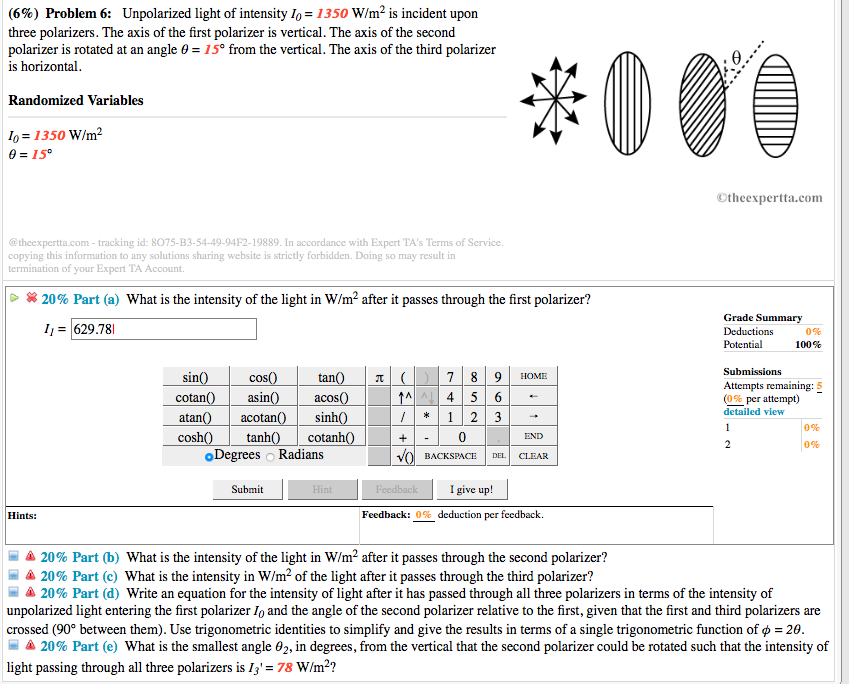(6%) Problem 6: Unpolarized light of intensity lo-1350 W/m2 s incident upon three polarizers. The axis of the first polarizer is vertical. The axis of the second polarizer is rotated at an angle θ-15° from the vertical. The axis of the third polarizer is horizontal Randomized Variables o 1350 W/m2 015° Otheexpertta.com @theexpertta.com-tracking id: 8075-83-54-49-94F2-19889. In accordance with Expert TA's Terms of Service. copying this information to any solutions sharing website is strictly forbidden. Doing so may result in termination...

• ### GOAL Understand how polarizing materials affect light intensity. Unpolarized light is incident upon three polarizers. The...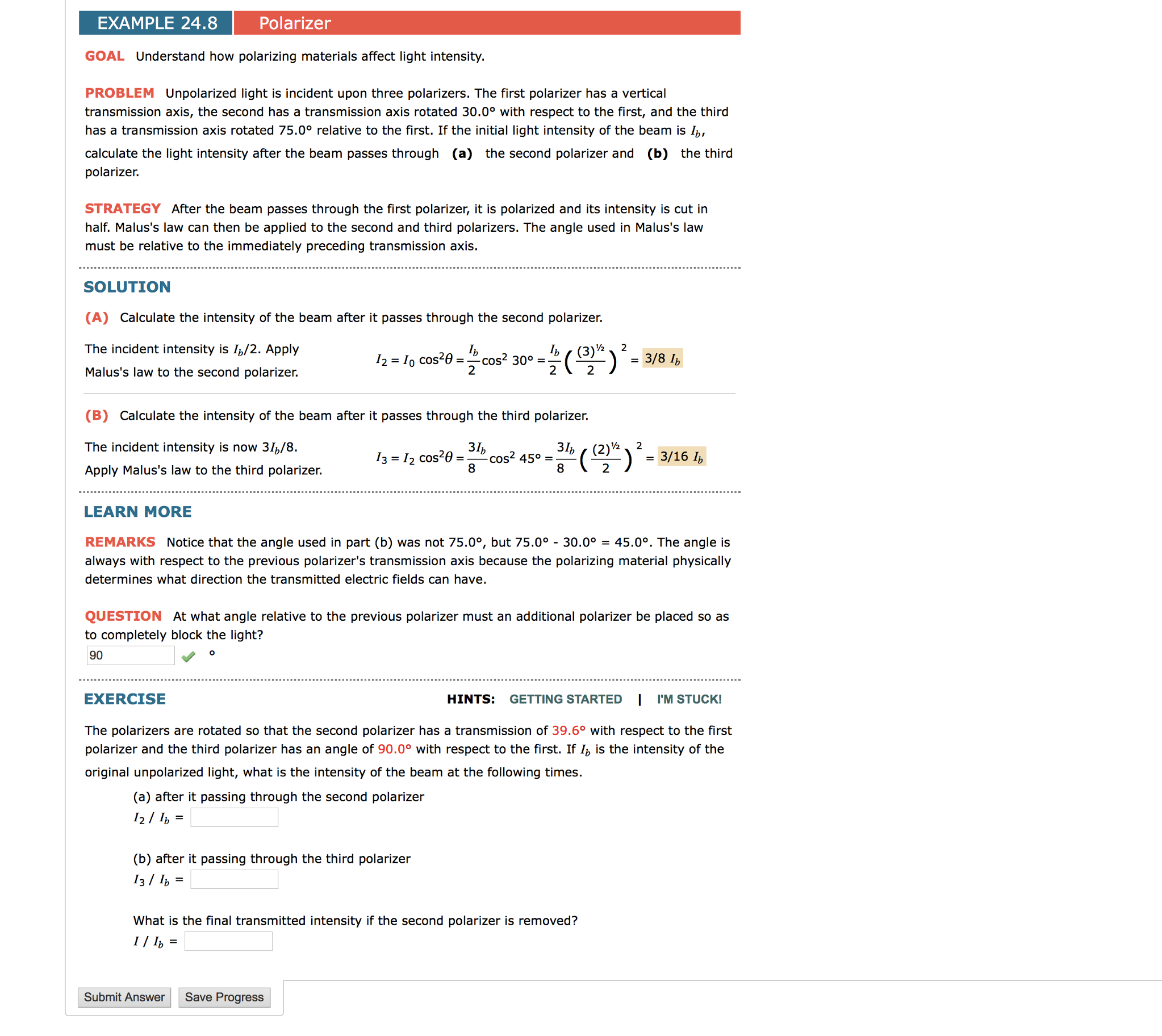GOAL Understand how polarizing materials affect light intensity. Unpolarized light is incident upon three polarizers. The first polarizer has a vertical transmission axis, the second has a transmission axis rotated 30.0 degree with respect to the first, and the third has a transmission axis rotated 75.0 degree relative to the first. If the initial light intensity of the beam is I_b, calculate the light intensity after the beam passes through the second polarizer and the third polarizer. After the beam...

• ### Light is entering a polarize or set of polarizers from the le Determine the elati e...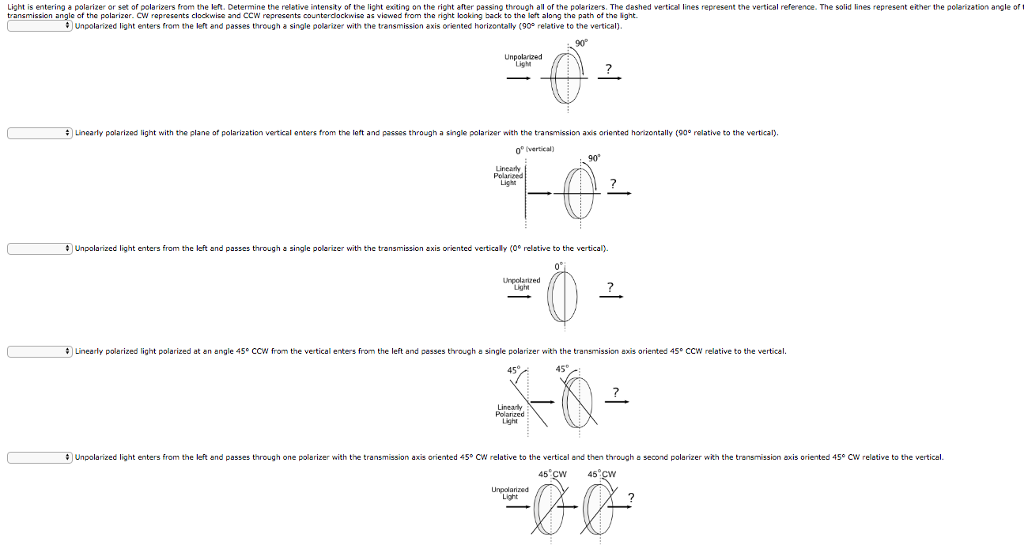Light is entering a polarize or set of polarizers from the le Determine the elati e intensity of the l??1 t exiting on the right after passing through al of the pole izers. The dashed vertical lines e resent the vertical e transmission angle of the polarizer. Crepresents clockwise and CCW represents countardockwise as viowed from the right loaking back to the left along the path of the light. ence. The solid lines represent either the ???? ization angle of...

• ### The diagram below shows a beam of light shining through three linear polarizers in a row. The polarizing axis of each polarizer is measured at an angle (ϴ1,ϴ2,ϴ3) from vertical. Suppose that the orig...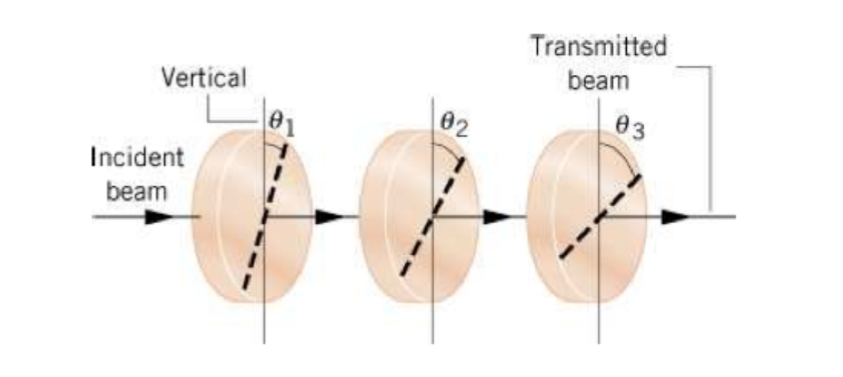The diagram below shows a beam of light shining through three linear polarizers in a row. The polarizing axis of each polarizer is measured at an angle (ϴ1,ϴ2,ϴ3) from vertical. Suppose that the original beam of light emanates from a vertically polarized laser. The laser has a power output of 1 mW and the beam has a diameter of 2 mm. What is the intensity of the laser beam before it travels through any polarizers? Calculate the final intensity of...

• ### please answer all 6 questions A monochromatic laser beam of intensity le=553 W/m2 is polarized in...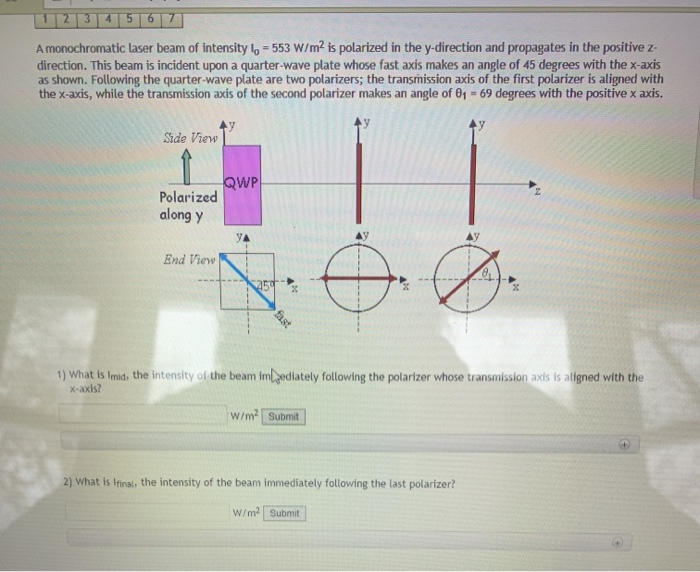please answer all 6 questions A monochromatic laser beam of intensity le=553 W/m2 is polarized in the y-direction and propagates in the positive z direction. This beam is incident upon a quarter-wave plate whose fast axis makes an angle of 45 degrees with the x-axis as shown. Following the quarter-wave plate are two polarizers; the transmission axis of the first polarizer is aligned with the x-axis, while the transmission axis of the second polarizer makes an angle of 01 =...

Free Homework App